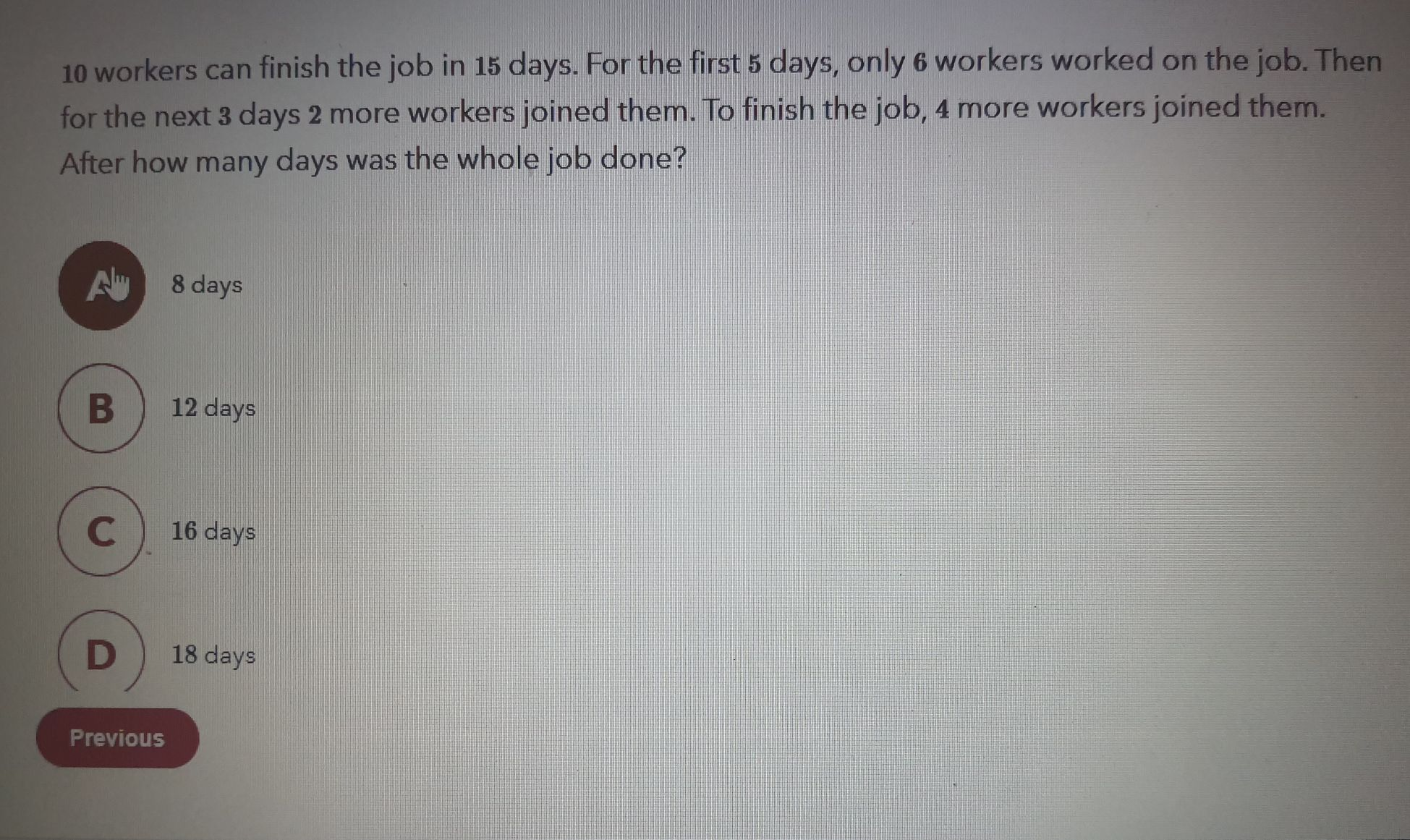### ¿Todavía tienes preguntas de matemáticas?

Pregunte a nuestros tutores expertos
Algebra
Pregunta$$10$$ workers can finish the job in $$15$$ days. For the first $$5$$ days, only $$6$$ workers worked on the job. Then for the next $$3$$ days $$2$$ more workers joined them. To finish the job, $$4$$ more workers joined them. After how many days was the whole job done? Previous $$12$$ days B days P $$18$$ days